## About & Pics

### Description

- With Geometrics, you are the creator, discoverer, artist, and mathematician.
- Advanced users can set the equation properties manually.
- Images can be saved to your device.

TRIGONOMETRY OVERVIEW:

A polar graph represents a math equation where the distance [r] from the center of the graph is determined by an equation. [r=1 is a circle. r=sin(angle) makes loops]
Polar Graph:
http://en.wikipedia.org/wiki/Polar_graph

Normally you start the angle from 0 and go all the way around to 360 degrees incrementing by some small amount. When you skip faster around the circle in a regular interval (say every 80 degrees), you get something interesting. A Maurer Rose http://en.wikipedia.org/wiki/Maurer_rose

Geometrics takes this maurer rose idea and puts you in control of the design:
The amount to skip, and a multiplier for the angle (to determine how many petals your maurer rose will have).

DESCRIPTION OF CONTROLS:

1. Swipe left or right - Restart the pattern changing the number of "flower petals"

2. Swiping up and down - Restart the pattern changing the "amount of skip"

3. Pressing and holding - Cycle through 3 trigonometric math functions (single sin equation, multiple sin equations, and a tan equation)

### Screenshots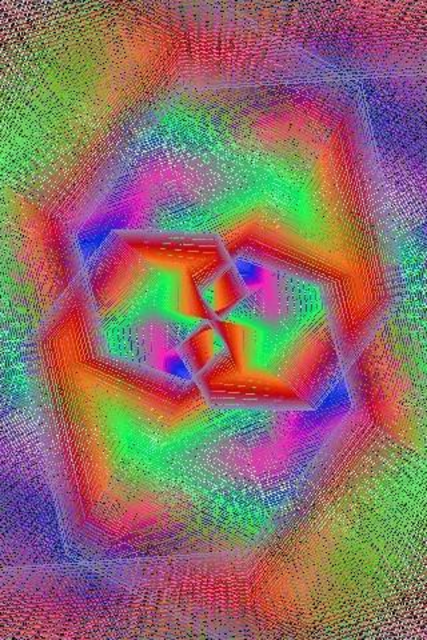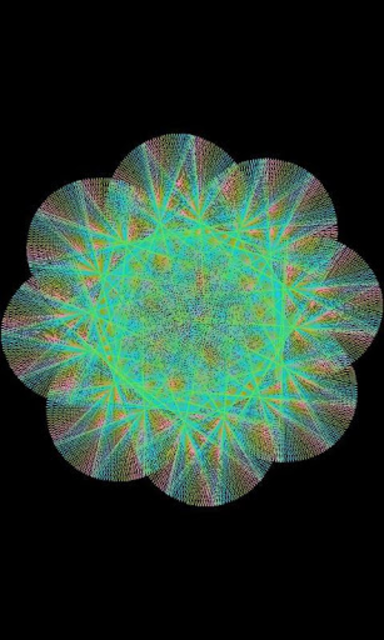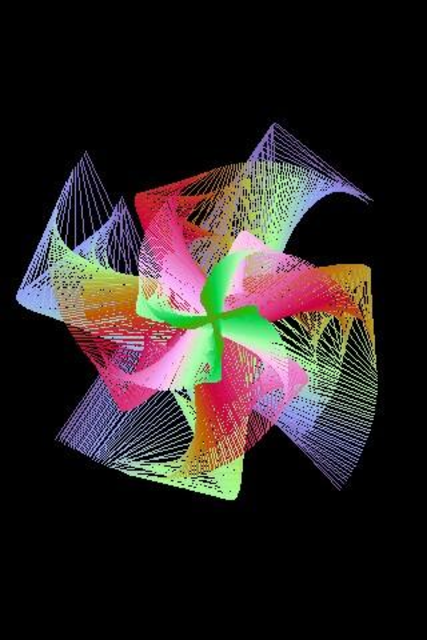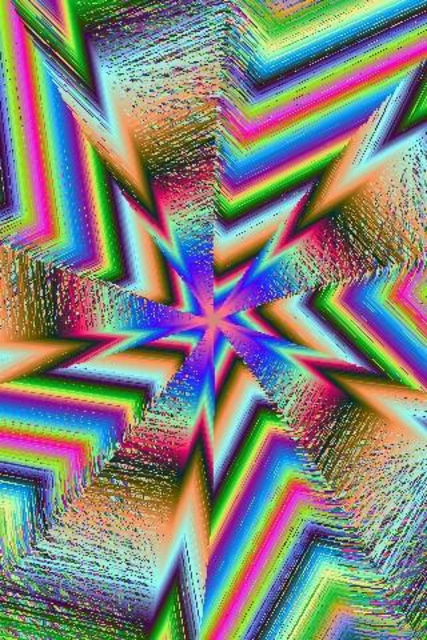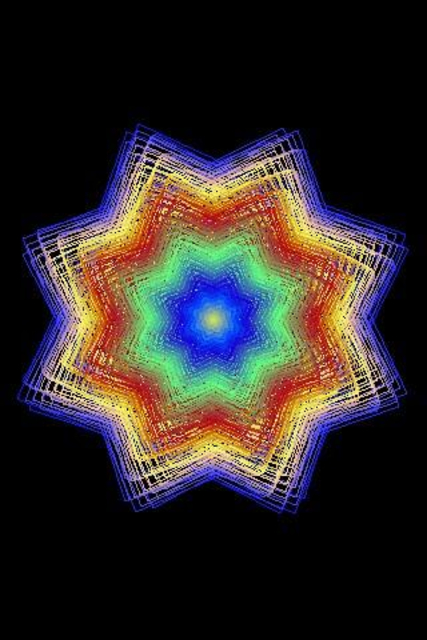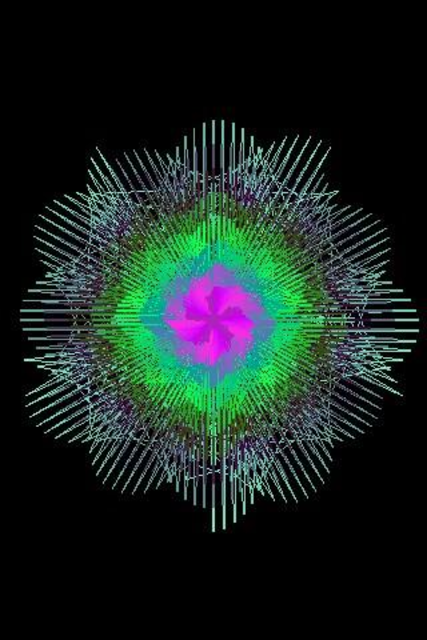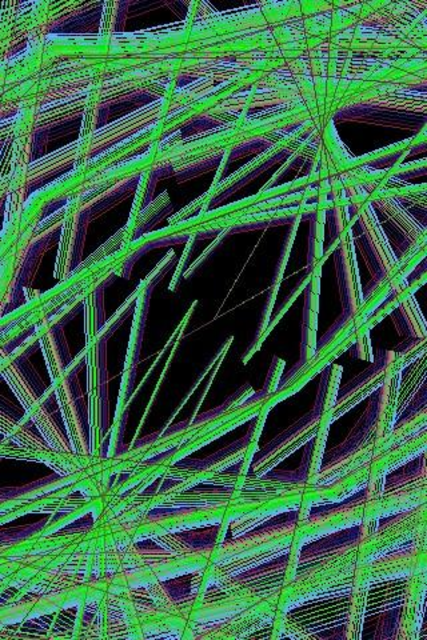### Version History

Launched Sep 05, 2013 (over 5 years ago).
 Sep 042013 (Current)Version 9FIXED: App can now save images to all devices.

### Ratings

This app has no ratings in the last 30 days.

8

### Total Ratings

 5 5 4 2 3 0 2 0 1 1